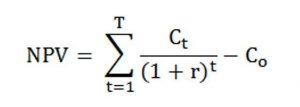Category: Finance KPIs.

The net present value is used to calculate the difference between the cash inflows and outflows of a potential investment or project. To make it easier to understand, it evaluates the profit generated by an investment in comparison with the cost set for the time value of money. Why this formula was invented? Because it shows that the value of a dollar could change from day to day.

And the opportunity cost and interest are the main reasons why such formulas are developed. The interest flow will always evaluate the future cash and it will cost more to borrow money. If you thought that economics is complicated, the below formula will definitely scare you away. However, there are much more simple ways to calculate the net present value.

Net Present Value (NPV) Formula

If you don’t know anything about math, you will do now. The below formula is complicated, so sit tight.To eliminate all doubts, the symbols represent the following values:

Ct
=
net cash inflow for the given period
t
=
number of periods
r
=
discount rate
CO
=
initial investment

It may look scary, but there is another way that you can calculate the NPV. By subtracting the present value of initial investment costs from the present value of future cash flows, you will get the NPV. The formula should look like this:

NPV
=
Present Value of Future Cash Flows – Present Value of Initial Investment Costs.

It definitely looks prettier than the above one, with not so many symbols involved.

Understanding the Net Present Value

Both formulas mentioned above are used by almost all types of investors. To get a better understanding of what NPV means, let’s think of a construction company. The management team wants to expand their business, but they don’t have the right equipment for this. So, the management uses the NPV formula to see if getting that equipment is a smart investment. It does so by comparing the cash inflows in the machinery that would benefit the company. Also, the price of the items is included in the calculation in the C0 value in the formula. If the outcome of the calculation is a positive number, then the cash that will come out of the purchase will be higher than the initial investment. However, if you get a negative number, then the cash will not make up for the money that was invested in the first place to buy the equipment.

Final Words

To conclude the above statements and information, taking the NPV as a prediction is the right thing to do. Basically, the Net Present Value is a kind of prediction like the weather forecasts are. The formulas are used to predict everything from the depreciation of money to the outcome of buying a piece of equipment and seeing if it is feasible. If the equipment is producing greater cash flow, that means that the investment is well made, so you will make up for the lost money in no time.

## Related Articles

• ### Sharpe Ratio

In order to be sound in your financial goals, you need to have a great source of knowledge on every... Read more